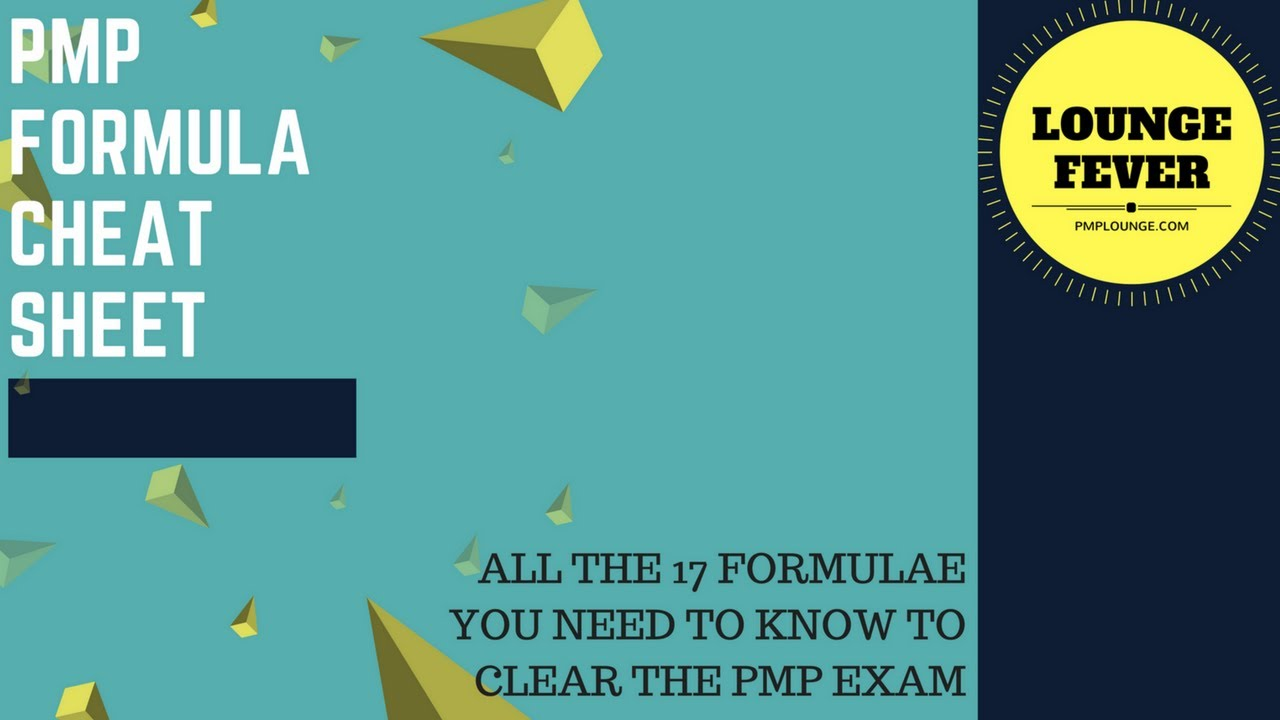PMP Formula Cheat Sheet – All the 16 Formulas you need to know to clear the PMP ExamMany participants worry about the mathematical questions in the PMP exam. We have created a PMP Formula cheat sheet for all those mathematical or numerical questions. The total number of formulas to be learnt are 16.

You can check out the video on YouTube here, alternatively all the formulas are listed below as well,

All PMP Formulas

1. No of Communication Channels = n(n-1)/2

2. SPI = EV/PV

3. CPI = EV/AC

4. SV = EV-PV

5. CV = EV-AC

6. EAC = AC+ETC

7. EAC = AC+BAC-EV

8. EAC = BAC/CPI

9. EAC = AC+(BAC-EV)/(CPI*SPI)

10. TCPI = (BAC-EV)/ (BAC-AC)

11. ETC = EAC-AC

12. VAC = BAC-EAC

13. PERT Estimation = (O + 4M + P)/6

14. STD DEVIATION = (P-O)/6

FLOAT SLACK

15. LS-ES

16. LF-EF

Now that you know all the formulas, don’t forget to check out the Common Names that you must learn as well.

PMP EXAM TIPS
https://goo.gl/97iZsK